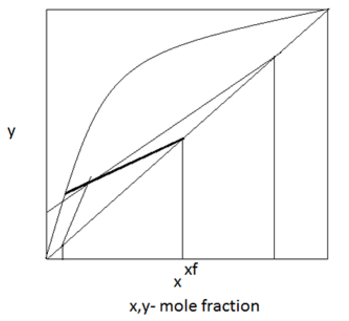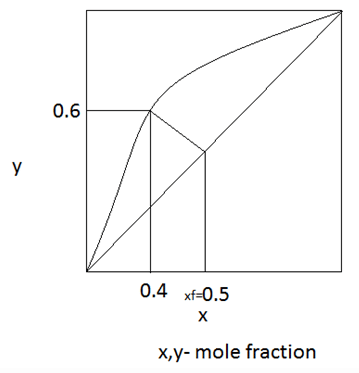# Test: Flash Vaporization Line (Q-line)

## 10 Questions MCQ Test Mass Transfer | Test: Flash Vaporization Line (Q-line)

Description
Attempt Test: Flash Vaporization Line (Q-line) | 10 questions in 20 minutes | Mock test for Chemical Engineering preparation | Free important questions MCQ to study Mass Transfer for Chemical Engineering Exam | Download free PDF with solutions
QUESTION: 1

### At what feed condition the slope of q line is infinity?

Solution:

Explanation: At saturated liquid the enthalpy of feed and the liquid is same so q=1 and slope = infinity.

QUESTION: 2

### Find the feed condition from the below distribution curve.

Solution:

Explanation: Since the slope of the q-line is between 1 and 0 it is a superheated vapour.

QUESTION: 3

### In an operation the slope of the q-line is found to be 0; then the enthalpy of feed equals enthalpy of _____________

Solution:

Explanation: Slope of q line = q/q-1
If the enthalpy of feed and vapour are equal then the q becomes zero; q line also becomes zero.

QUESTION: 4

Consider a saturated liquid-vapour mixture, if the liquid flow rate inside the enriching and the stripping section of a fractionator is 50 and 40 mol/hr. Also the feed rate is 10 mol/hr. Find the feed condition.

Solution:

Explanation: q= 50-40/10 = 1; then the feed condition is a saturated liquid.

QUESTION: 5

Find the liquid vapour compositions.Solution:

Explanation: From the feed condition we can estimate that the feed has a mixture of liquid and vapour. From the distribution diagram, the vapour composition is 60% and the liquid composition is 40%.

QUESTION: 6

At what feed condition the slope of the flash vaporization line becomes 0?

Solution:

Explanation: For a saturated vapour, the enthalpy of feed and the enthalpy of vapour is same so the value of q= 0; slope of q-line is 0.

QUESTION: 7

The point where the operating line and the equilibrium line meets in distillation methods is known as

Solution:

Explanation: At the pinch point, separation is not possible since it requires infinite number of trays in the enriching section if operating and equilibrium line meets.

QUESTION: 8

Find the exact q line for the saturated liquid from the distribution diagram,Solution:

Explanation: For the saturated liquid, slope of q-line should be infinity.

QUESTION: 9

The equilibrium composition of liquid and vapour in a feed can be identified by,

Solution:

Explanation: If the value of q is between 0 and 1 then the feed has both vapour and liquid. Then the compositions can be identified by intersecting q-line with operating line.

QUESTION: 10

If the feed enters the distillation column at its dew point, then the slope of the Flash vaporization operating line is

Solution:

Explanation: If the feed at dew point means the feed condition is saturated vapour so the enthalpy of feed is same as the enthalpy of the vapour. Therefore the slope of the Q-line is 0. Since Q-line is the Flash vaporization operating line.Use Code STAYHOME200 and get INR 200 additional OFF Use Coupon Code